Courses

# Alphanumeric Series, Introduction and Examples (with Solutions), Reasoning (Part - 1) LR Notes | EduRev

## Banking Exams : Alphanumeric Series, Introduction and Examples (with Solutions), Reasoning (Part - 1) LR Notes | EduRev

The document Alphanumeric Series, Introduction and Examples (with Solutions), Reasoning (Part - 1) LR Notes | EduRev is a part of the Banking Exams Course Reasoning Aptitude for Competitive Examinations.
All you need of Banking Exams at this link: Banking Exams

Alphanumeric (sometimes shortened to alphameric) is a combination of alphabetic and numeric characters. In some cases, it may include upper and lower case letters, punctuation marks, and symbols (such as @, &, and *).

This type of reasoning is a mixed bag of coding-decoding, series-based reasoning and finding positions based reasoning questions.

Type of question

1. Immediately Preceded By
2. Immediately Followed By
3. Based on word/ Number / symbol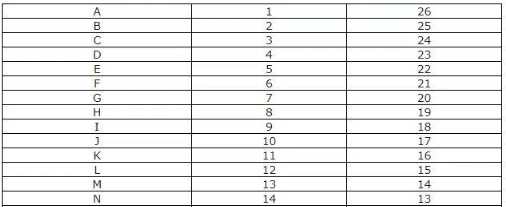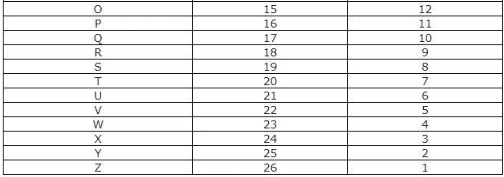Example 1: Study it carefully and answer the questions that follow:
L F 3 # R N 8 A @ Y 4 M © W P 6 H U 9 I K 2 E

Q. If all numbers are dropped from the above arrangement, which of the following will be the fourteenth from the left end?
a) I
b) P
c) W
d) U
e) None of these

If all number are dropped, the arrangement will look like-

L F # R N A @ Y M © W P H U I K E

ATQ, 14th From Left end -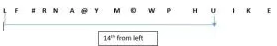Example 2: Study the following arrangement carefully and answer the questions given below.

R 4 P I J M Q 3 % T @ © U K 5 V 1 W \$ Y 2 B E 6 # 9 D H 8 G & Z N

Q. How many such numbers are there in the above arrangement each of which is immediately preceded by a vowel?
a) None
b) One
c) Two
d) Three
e) None of these

STEP 1) 1st we have to identify all the vowel in the arrangement.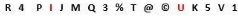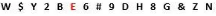Vowel in Above arrangement: I, U, E

STEP 2) ATQ, numbers in the above arrangement each of which is immediately preceded by a vowel.

Since we can see there is no such number which is preceded by vowel.

Example 3: C2E, E5H, G12K, 127N, ____?

(a) I58P
(b) J58Q
(c) K58Q
(d) I57Q

Solution:
For Numbers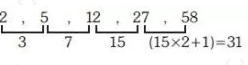For Alphabet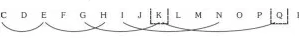So option (c) K58Q.

Practice Problems On Alphanumeric Series

Directions (1-5): Study the following arrangement and answer questions given:

( F E ~ 6 2 % S A 8 B ) * 1 L O 9 @ W U \$ 3 ^ P 4 C V #

Q.1. How many such vowels are there in the above arrangement, each of which is immediately followed by a symbol and not preceded by a letter?

a) Four

b) Three

c) Two

d) One

e) None

Solution: Option (e)

Q.2. What is the sum of all numbers which are immediately preceded by a symbol?

a) There are no such numbers

b) 10

c) 12

d) 9

e) 11

Solution: Option (b)
Explanation:
6+1+3 = 10

Q.3. What will come in place of questions mark (?) based on the above arrangement?

3U^  \$W3  U@\$  W9U ?

a) 9OW

b) @9W

c) @OW

d) U@W

Solution: Option (c)

Q.4. Four of the following five are alike in a certain way based on their positions in the above arrangement and so form a group. Which is the one that does not belong to that group?

a) (#V

b) EC4

c) 2^P

d) S\$U

e) *OL

Solution: Option (c)

Q.5. Which of the following is the 9th to the left of the 17th from the left end of the above arrangement?
a) @
b) S

c) 9

d) %

e) O

Solution: Option (b)
Explanation:
Left of left:- 17-9 = 8th from left = S

Directions (6-10): Study the following arrangement and answer questions given:

K J 2 ^ U ! A S 3 C 7 \$ 9 L # D O 8 X I % N P 4 E * M + W

Q.6. How many such numbers are there in the above arrangement, each of which is immediately preceded by a consonant and immediately followed by a vowel?
a) One
b) Three
c) Two
d) Five
e) None

Solution: Option (a)
Explanation: Only 4 — P 4 E

Q.7. How many such consonants are there in the above arrangement, each of which is immediately preceded by a symbol and immediately followed by a letter?
a) Four
b) Three
c) Two
d) Five
e) None

Solution: Option (c)
Explanation: D, N

Q.8. Which of the following is the 8th to the right of the 19th from the right end of the above arrangement?
a) %
b) #
c) 8
d) X
e) None of these

Option D
Explanation:
Right to right = 19-8 =11th from right = X

Q.9. Four of the following five are alike in a certain way based on their positions in the above arrangement and so form a group. Which is the one that does not belong to that group?
a) \$97
b) U!^
c) XI8
d) +WM
e) C7S

Solution: Option (e)

10.Which of the following is the 5th to the left of the 13th from the right end of the above arrangement?
a) 7
b) 9
c) S
d) \$
e) !

Solution: Option (d)
Explanation: Left of right = 13+5 =18th from right

Directions (11-15): In each question below is given a group of letters followed by four combinations of digits/symbols given in (A), (B), (C) and (D). You have to find out which of the combinations correctly represents the group of digits based on the rules and mark the answer accordingly. If none of the combinations correctly represents the group of letters, mark (E) as your answer.

(i) If the first two letters are vowels, the codes for first and last letters are to be interchanged.
(ii) If the first letter is a consonant, the codes for the second and third letters are to be interchanged.
(iii) If the last letter is a vowel then the second letter is to be coded as the code for the vowel.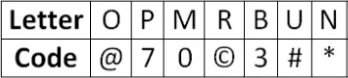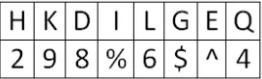Q.11. UEPMOQ
a) 3^70@#
b) 4^70!#
c) 4^70@#
e) None of these

Solution: Option (c)
Explanation: The given number satisfies rule (i)

Q.12. OPHDLU
a) #@286#
b) @#286#
c) @#246
d) @#286
e) None of these

Solution: Option (b)
Explanation: The given number satisfies rule (iii)

Q.13. MIRKNQ
e) None of these

Solution: Option (c)
Explanation: The given number satisfies rule (ii)

Q.14. HOLUIB
a) 26@!%3
b) 26^#%3
c) 26@#%7
d) 24@#%3
e) None of these

Solution: Option (e)
Explanation:
The given number satisfies rule (ii)
26@#%3

Q.15. OINRBK
e) None of these

Solution: Option (d)
Explanation: The given number satisfies rule (i)

Directions (16-20): Study the following arrangement and answer questions given:

4 8 @ B D E ! Y I 7 * K W 6 A L 5 # 9 O 2 U ^ © 3 N ( M \$

Q.16. Which of the following is the 9th letter to the left of the 16th letter from the right end?
a) E
b) B
c) D
d) *
e) @

Solution: Option (c)
Explanation: Left to right = 16+9 = 25th from right

Q.17. How many such letters are there in the above arrangement each of which is immediately preceded by a symbol and immediately followed by a consonant?
a) Four
b) Three
c) None
d) One
e) Five

Solution: Option (b)
Explanation:
B, K, and M

Q,.18. Complete the series: 4\$V (BD !©^ 27* ?
a) K#5
b) W95
c) W#5
d) W9#
e) K9#

Solution: Option (c)
Explanation:

• 4\$V – 4=first element, \$= first element from last, V = previous letter of \$
• (BD – ( = leaving one letter from V, ( = 4th element from last, B= 4th element from starting, D = next letter to B
• !©^ – ! = leaving one letter from D, ! = 7th from starting, © = 7th from last, ^ = previous to ©
• 27* – 2 = leaving one letter from ^, 2 = 10th from last, 7 = 10th from starting, * = next to 7

Q.19. How many such numbers are there each of which is immediately preceded by a symbol?
a) One
b) Two
c) Three
d) More than three

Solution: Option (b)
Explanation: 9, and 3

Q.20. Four of the five are alike in a certain way based on arrangement, which does not belong to the group?
a) KW*
b) BD@
c) MV(
d) V\$(

Solution: Option (d)

Offer running on EduRev: Apply code STAYHOME200 to get INR 200 off on our premium plan EduRev Infinity!

## Reasoning Aptitude for Competitive Examinations

7 videos|21 docs|110 tests

,

,

,

,

,

,

,

,

,

,

,

,

,

,

,

,

,

,

,

,

,

,

,

,

,

,

,

;